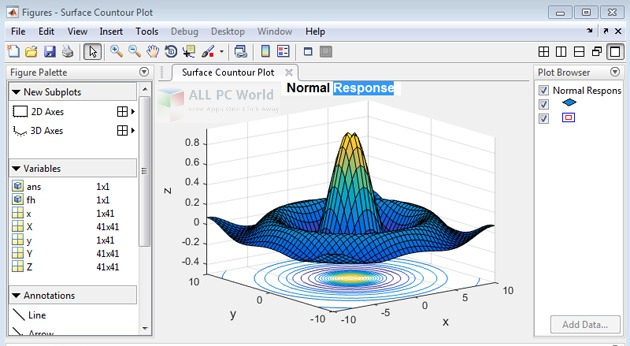Download MathWorks MATLAB R2015a free setup for windows. This MATLAB R2015a is a complete simulation package for engineering applications that deal with designing electrical and mechanical control systems.

## MathWorks MATLAB R2015a Overview

MathWorks MATLAB R2015a is a high-level language and interactive environment for numerical computation, visualization, and programming of electrical and mechanical control systems. It offers an intuitive and graphical user interface for analyzing data, developing algorithms, and creating models and applications. MATLAB R2015a is fully loaded with the latest drawing tools and built-in math functions that help the engineers and designers to explore multiple approaches and reach a solution faster than with spreadsheets or traditional programming languages, such as C/C++ or Java.The version MATLAB R2015a includes four new products Antenna Toolbox, Robotics System Toolbox, Simulink Test, and Vision HDL Toolbox. The Antenna Toolbox is for designing, analyzing, and visualizing antenna elements and antenna arrays while Robotics System Toolbox deals with designing and testing algorithms for robotics applications.  The other two products Simulink Test is specially used for creating test harnesses, authoring complex test sequences, and managing simulation-based tests while the Vision HDL Toolbox is for designing image processing, video, and computer vision systems for FPGAs and ASICs.

## Features of MathWorks MATLAB R2015a

• Complete simulation package for engineering applications
• Integration of documentation for custom toolboxes
• Enhanced design environment and expanded UI component
• Support for Arduino Leonardo and other Arduino boards
• Image acquisition toolbox and image processing toolbox
• Plot with date time and categorical data
• Define local functions in scripts for improved code

## System Requirements for MathWorks MATLAB R2015a

• Operating Systems (win 7, win 8, win 8.1 and win 10)
• Installed Memory (RAM):  4 GB Recommended For Excellent Performance
• 4 GB HDD
• File Name: Mathworks_Matlab_R2015a_32bit.zip, Mathworks_Matlab_R2015a_64bit.zip